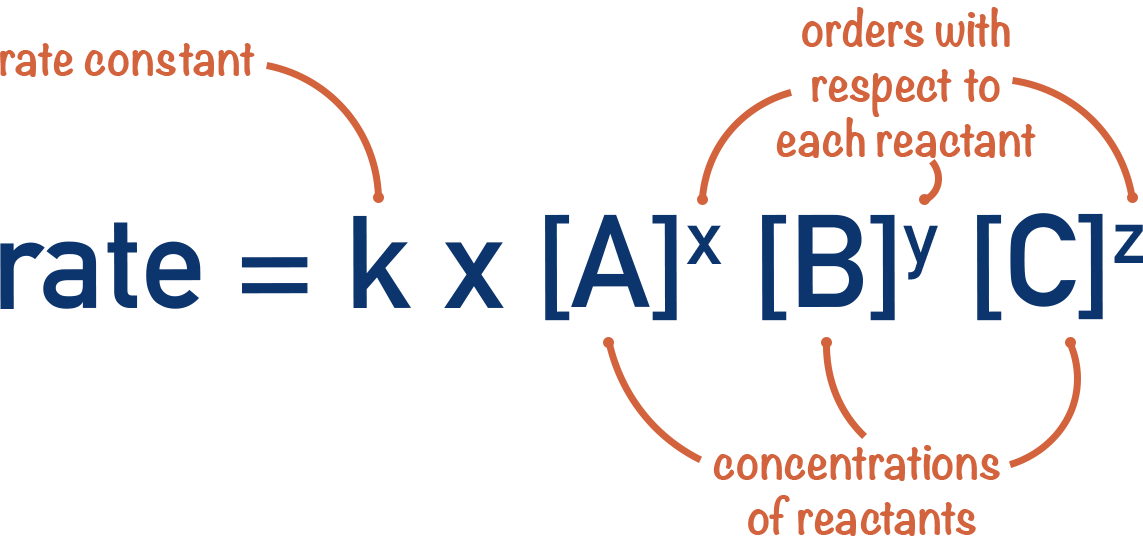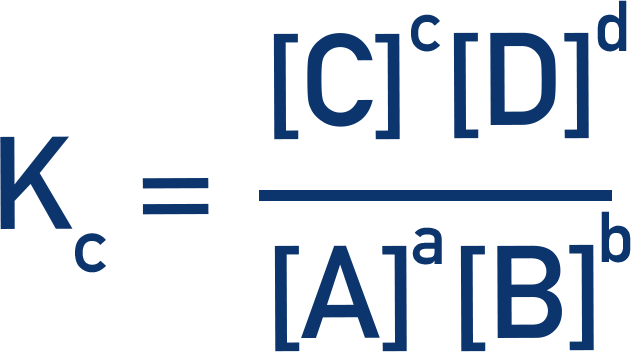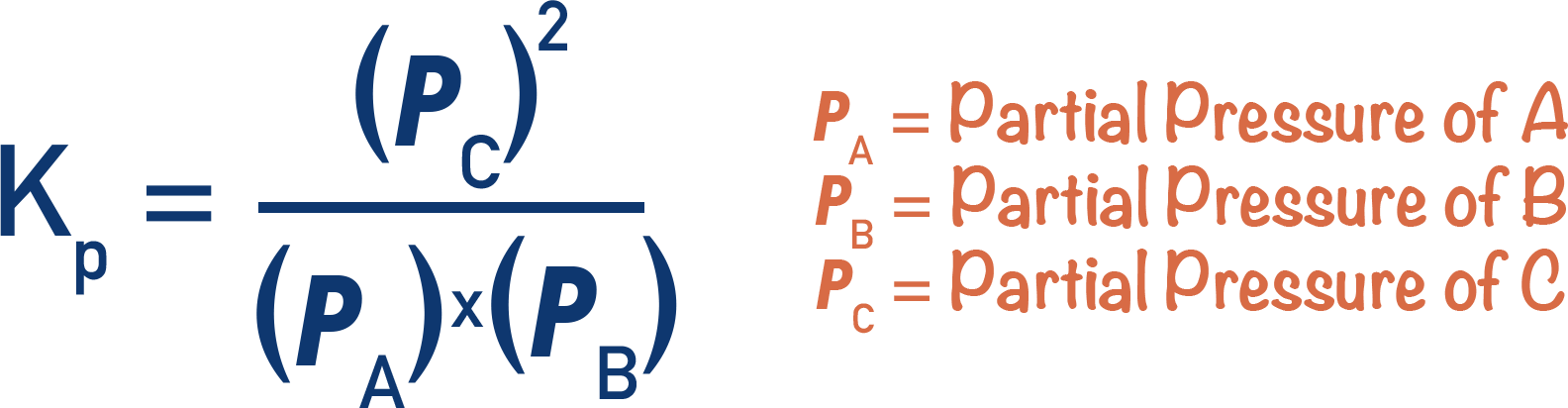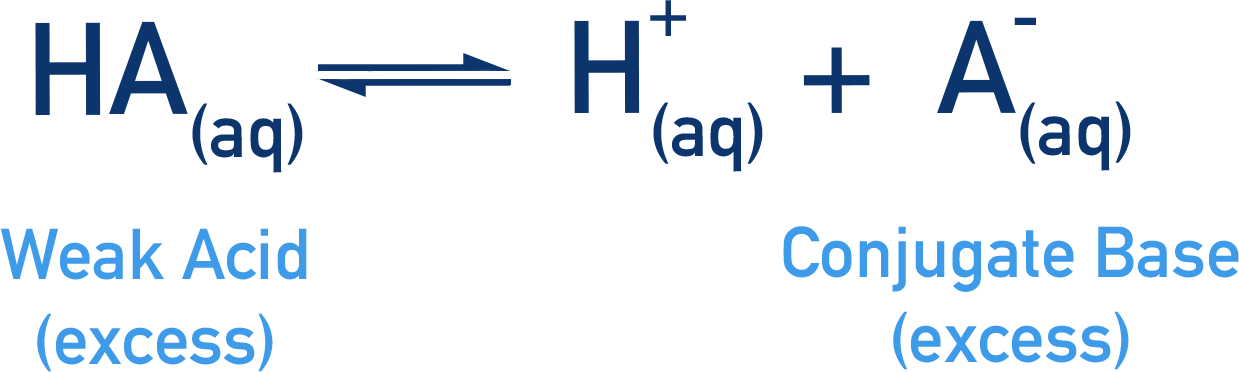# Energy and Entropy (Thermodynamics)

Lattice Enthalpies

• Lattice enthalpy of formation is the enthalpy change that occurs when 1 mole’s worth of an ionic compound is formed from its constituent, gaseous ions.
• Lattice formation enthalpies are always negative (exothermic process).
• Lattice enthalpy of dissociation is the enthalpy change that occurs when 1 mole’s worth of an ionic lattice is broken apart into its constituent, gaseous ions.
• Lattice dissociation enthalpies are always positive (endothermic process).
• Solid ionic lattices are held together by strong electrostatic attraction between oppositely charged ions.
• Ion charge and size influence the strength of ionic attraction.
• The stronger the attraction between ions, the stronger the lattice (higher melting point).
• When two opposite charges come close together their energies lower, releasing energy to the surroundings.

Born-Haber Cycles

• Born-Haber cycles are used to find lattice enthalpies.
• It is very difficult to determine lattice enthalpies experimentally, this is why Born-Haber cycles are used.
• Hess’ Law states that the overall energy change that occurs when a product is formed from its constituent elements is the same, regardless of the route taken to form the product (at the same temperature and pressure).
• Born-Haber cycles are made by using two different routes to form an ionic lattice from its constituent elements in their standard sates.
• Different standard reactions are used to construct a Born-Haber cycle.
• Standard enthalpy of atomisation – enthalpy change when one mole of gaseous atoms is formed from its element (in its standard state).
• (First) Ionisation energies – enthalpy change when one electron is removed from each atom in one mole’s worth of gaseous atoms.
• (First) Electron affinities – enthalpy change when one electron is added to each atom in one mole’s worth of gaseous atoms.
• Standard enthalpy of formation – enthalpy change when one mole of a compound is formed from constituent elements in standard states.

Entropy

• Entropy is a measure of disorder within a system.
• If there are lots of possible of ways of arranging particles in a system, it has a higher entropy.
• If there are fewer possible ways of arranging particles in a system, it has a lower entropy.
• Solids have low entropies; gases have high entropies. Going from solid to gas increases the entropy of a system as there are then more possible ways to arrange the particles.

Entropy Change

• Entropy change, ΔS = (sum of standard entropies of products) – (sum of standard entropies of reactants)
• Standard entropies, S, enable the entropies of different substances to be compared and the overall entropy change that occurs in a reaction can be found.
• The standard entropy of a substance refers to the entropy of 1 mole of the substance, measured at standard conditions (298K and 101kPa).
• Units for standard entropy are JK-1mol-1
• A substance has different entropies depending upon its state and conditions.
• Water has a low entropy below 0oC (as it’s a solid), but a high entropy at over 100oC (as it’s a gas).

Free Energy (Gibbs)

• The feasibility of a reaction refers to how likely it is to happen.
• High feasibility means a reaction is likely to happen.
• Low feasibility means a reaction is unlikely to happen.
• For a reaction to be feasible, energy must be released overall.
• quick notes
• The overall energy change of a reaction is determined by both the change in enthalpy and the change in entropy that would occur.
• Gibbs free energy (ΔG) is a value calculated to shows how energy changes overall during a reaction.
• The equation is: ΔG = ΔH - TΔS
where ΔH = enthalpy change, T = temperature (in Kelvin) and ΔS = entropy change.
• If Gibbs free energy is negative, the reaction is feasible.
• If Gibbs free energy is positive, the reaction is not feasible.
• If for a reaction:
• -ΔH and +ΔS, the reaction is always feasible.
• -ΔH and -ΔS, the reaction may be feasible at low temperatures.
• +ΔH and +ΔS, the reaction may be feasible at high temperatures.
• +ΔH and -ΔS, the reaction is never feasible.

Total Entropy

• The total entropy change that occurs in a reaction can be calculated using:• During a chemical reaction, the entropy of reacting particles and their surroundings change.
• How the entropies of the reacting particles change in a reaction is shown by entropy change of the system, ΔSsystem.
• How the entropy of the surroundings changes in a reaction is shown by entropy change of the surroundings, ΔSsurroundings.
• The entropy change of the system and the entropy change of the surroundings can be combined to describe the total entropy change of the reaction, ΔStotal.
• The entropy of the surroundings is linked to the temperature of the reaction and the enthalpy change that occurs during the reaction.
• It can be calculated by dividing the change in enthalpy (ΔH) by the temperature (T).
• For a reaction to be possible, its change in total entropy must be positive (+ΔStotal).

# Rates of Reaction (Kinetics)

Rates of Reaction

• How fast a reaction happens is called its rate of reaction and is measured with the units mol dm-3 s-1.
• Rates of reaction can only be found experimentally by measuring a change in concentration of a reactant or product in a given amount of time.
• Concentration of reactants, temperature of the reaction, the pressure of a (gaseous) system and the activation energy for a reaction all determine the rate of a reaction.
• Kinetics is the study of reaction rates (how quickly reactions occur) and the factors that control the rate of a reaction.

Orders of Reaction

• Changing the concentration of reactants in a reaction can affect the rate of the reaction.
• The orders of a reaction describe how much the rate of a reaction changes when the concentrations of reactants are changed.
• The order of a reaction with respect to a reactant describes how much the rate of a reaction changes when the concentration of that reactant is changed by a given factor.
• Zero order means changing the concentration of the reactant has no change on the rate of reaction.
• First order means changing the concentration of the reactant by a given factor will change the rate of the reaction by the same factor.
• Second order means changing the concentration of the reactant by a given factor will change the rate of the reaction by the same factor squared.

The Rate Equation

• A rate equation links the rate of a reaction at a particular temperate to the concentrations of all reactants.
• A rate constant, k, is used in the rate equation to account for the influence of temperature.
• A rate constant is only for a given temperature.
• Different reactions can have different rate constant values and the units can also be different.
• In the rate equation, each concentration of reactant is raised to the power of its order and multiplied by the rate constant, k.Clock Reactions

• Clock reactions are used to find the initial rate of a reaction.
• The length of time taken to form a small amount of product is measured.
• A colour change or observation is used to show when this small amount of product has formed.
• Measuring the initial rate of a reaction is difficult because the concentrations of the reactants are constantly changing, meaning the rate of reaction is also changing.
• The iodine clock experiment is a common example of a clock reaction at A-level Chemistry.
• In the main reaction, hydrogen peroxide reacts with iodide ions to produce iodine molecules.• In a second reaction, thiosulfate ions react with the iodine produced in the main reaction to form iodide ions again.• Starch indicator is added to the reaction mixture, which turns dark blue in the presence of iodine. As long as there are thiosulfate ions in the mixture, the starch will not cause a colour change.
• The thiosulfate ions are instantly converting any iodine molecules formed into iodide ions.
• When all the thiosulfate ions are used up the starch will cause a colour change.
• Iodine molecules will no longer be reacted back to iodide ions by the thiosulfate ions and they can react with the starch (causing colour change).
• The amount of thiosulfate ions in the mixture is known and the length of time taken for the mixture to change colour is recorded.
• This is used to find how long it took to form a specific amount of iodine and give the initial rate of reaction.

# Equilibrium

Equilibrium Constant

• Equilibrium of a reversible reaction is achieved when the concentrations of both the reactants and products remain constant.
• At equilibrium, the rate of the forward reaction is the same as the rate of the backward reaction.
• The ‘position of equilibrium’ describes how much either the forward or reverse reaction was favoured before equilibrium was reached.
• This can be shown mathematically by comparing the concentrations of reactants to products at equilibrium.
• The equilibrium constant, Kc, is a constant that describes the ratio between reactants and products at equilibrium.
• It is calculated by dividing the concentrations of the products by the concentrations of the reactants (raised to the power of their molar ratios).• Kc values are only for an equilibrium at a specific temperature, if the temperature of an equilibrium system is changed, the value of Kc will also change.

Moles Fractions and Partial Pressures

• How much pressure one type of gas contributes to the total pressure of a gaseous system is called its partial pressure.
• All partial pressures of gases in a system add up to give the total pressure of the system.
• The mole fraction of a type of gas describes how many moles of that gas there are compared to all other gases in the system.
• The total pressure of a gaseous system is directly related to the number of moles of all gases in the system.
• 1 mole of all gases are assumed to occupy the same volume in space (24 000cm3 at room temperature and pressure).

Equilibrium Constant, Kp

• The equilibrium constant, Kp, describes the ratio of reactants compared to products at equilibrium for a gaseous equilibrium system.
• Kp is found by dividing the partial pressures of all products by the partial pressures of all reactants (raised to the power of their molar ratios).• The partial pressures of a gas in a closed system are directly linked to the moles of that gas.
• Partial pressures of reactants and products can be used to determine how much the forward or reverse reaction is favoured.

# Electrochemistry

Electrochemistry - The Basics

• Metals react by losing electrons and forming positively charged ions, this is oxidation.
• The reactivity of a metal refers to how easily it can lose electrons (and form positively charged ions).
• Electrodes are solid surfaces that, when in solution, can ‘hold’ electrons on their surface or give electrons away.
• Simple half-cells are made of a metal electrode placed into an electrolyte, which contains ions of the metal used for the electrode.
• When a reactive metal electrode is placed in an electrolyte containing ions of itself, an equilibrium is established between the ions in the electrolyte and the metal electrode.
• A build-up of electrons on an electrode’s surface creates an electrical potential.
• Charged ions cause the electrolyte to have an electrical potential.
• The electrical potential of the electrode and the electrical potential of the electrolyte are different – creating a potential difference between the two – this is called an electrode potential.
• Different metals have different electrode potentials based on their reactivities.

Electrochemical Cells

• If two different half-cells are connected by a wire (and a salt bridge), an electrical current is created and electricity flows.
• This is called an electrochemical cell.
• The half-cell with the most electrons on its electrode (more negative electrode potential) will force its electrons to flow to the half-cell with fewer electrons on its electrode (more positive electrode potential).
• The potential difference between the two connected electrodes can be measured using a voltmeter.
• The greater the difference in potential (voltage) between the two half-cells, the greater the electrical current produced when they are connected.
• Electrical current is the net movement of charge in a direction.
• Electrons have a charge, so if there’s a net movement of electrons in one direction, an electrical current is produced.
• It’s the relative difference between potentials that’s important in electrochemistry.
• It’s not possible to measure the actual potential of a half-cell, just how different it is compared to other half-cells.

Electrode Potentials

• The relative potentials of half-cells are found by comparing them all to the same type of half-cell. The half-cell used is called the standard hydrogen electrode.
• If two hydrogen half-cells (standard hydrogen electrodes) are connected, the potential difference of the electrochemical cell = 0V.
• If one of the half-cells is changed to a different type of half-cell, there will be a difference in potential between the two – enabling a comparison to be made.
• All potential differences measured are referred to as standard electrode potentials.
• For an electrode potential to be considered standard, all ions in solution for the half-cell being used must be 1 mol dm-3, temperature must be 298K and, for gaseous half-cells, the pressure must be 101kPa (standard conditions).
• The potential difference for a cell (Ecell) made of two different half-cells can be calculated by finding the difference between their standard electrode potentials.• In an electrochemical cell the cathode is the electrode where reduction takes place, the anode is the electrode where oxidation takes place. So, finding the Ecell potential difference can also be written as:Electromotive Force, EMF

• In an electrochemical cell, charge flows between two electrodes because of a potential difference between them.
• Once both potentials are the same, no charge will flow.
• To maintain a constant flow of charge, the potential difference between the electrodes needs to be maintained – this requires energy.
• The amount of energy needed to maintain a certain potential difference is called the electromotive force (EMF).
• The electromotive force in electrochemical cells comes from the energy released by redox reactions occurring in each half-cell, keeping the potentials different.
• As reactants are used up, the reactions slow down meaning the electromotive force drops, resulting in less potential difference between electrodes, so a smaller current is produced. This is why batteries lose power and ‘go flat’.

# Acids and Bases

Acids and Bases

• Acids are species that donate protons and bases are species that accept protons.
• This is the Bronsted-Lowry definition of acids and bases.
• In solution, acids ‘dissociate’ (split apart) and release protons to water molecules, causing hydroxonium ions (H3O+) to form.
• Hydroxonium ions can react with bases, giving the extra proton to the base and reforming water.
• In equations, H+(aq)is used to represent a proton.
• The proton actually exists in solution with water as hydroxonium ions (H3O+).
• Acids lose protons to form their conjugate base.
• Bases gain protons to form their conjugate acid.

Strong and Weak Acids

• Strong acids fully dissociate in solution – all molecules of the acid split, releasing the maximum number of protons, H+(aq), possible.
• Weak acids partially dissociate in solution – some acid molecules that have released a proton re-gain a proton to form a molecule of the acid again.
• Monoprotic acids release one H+(aq) ion for every one molecule of acid.
• Diprotic acids release two H+(aq) ions for every one molecule of acid.
• The concentration of a strong, monoprotic acid solution is the same as the concentration of H+(aq) ions present in the solution.

Acid Dissociation Constant, Ka

• Weak acids only partially dissociate in solution, forming an equilibrium between the acid and its conjugate base.• Acid dissociation constants, Ka, are used to show how much either the forward or reverse reaction is favoured and to give an indication of the acid’s strength.
• If a weak acid is shown as HA and its conjugate base as A-, then the Ka for that acid can be found using:• The greater the value of Ka, the stronger the acid.
• The smaller the value of Ka, the weaker the acid.

pH Calculations

• pH (potential of hydrogen) is a scale used to show how acidic or alkaline a solution is.
• Acidity is determined by the concentration of H+(aq) ions in a solution, meaning pH is a way of describing the H+(aq) concentration in a solution.
• The pH scale is logarithmic, meaning a change in pH value of one refers to a change in concentration of H+(aq) ions by a factor of 10. It can be calculated using:

pH = - log10[H+]

[H+] = 10-pH

• pH of 0 means a solution has a H+(aq)ion concentration of 1 mol dm-3.
• pH of 1 means a solution has a H+(aq) ion concentration of 0.1 mol dm-3.

Ionic Product of Water, Kw

• The ionic product of water, Kw, is a constant that links the concentration of OH-(aq) ions in solution to the concentration of H+(aq) ions in solution.• To find the pH of an alkaline solution, the concentration of H+(aq) ions in solution can be found using the ionic product of water, Kw.• At 298K, Kw has a fixed value (1 x 10-14).

Buffer Solutions

• Buffer solutions contain a mixture of a weak acid and its conjugate base.
• They minimise a change in pH of a solution when H+(aq) ions are added or ‘removed’ (by addition of a base or alkali).
• An equilibrium is established between the weak acid and conjugate base.• The weak acid and conjugate base are in excess, meaning that the position of the equilibrium established between them will not be sensitive to changes in their concentrations, but it will be very sensitive to changes in the concentration of H+(aq) ions.
• When H+(aq) ion concentration is increased or decreased, the position of equilibrium moves to oppose the change.

Titration Curves

• Titrations are commonly used to find the unknown concentration of an acid (or base) by reacting it perfectly with a base (or acid) of known concentration.
• The volume of ‘known’ concentration solution added is called a titre and is used to determine the moles of acid or base in the volume of unknown concentration used in the titration.
• Titration curves show how the pH of an acidic or basic solution changes as a basic or acidic solution is added to it.
• The end point of a titration is the point at which an indicator changes colour at a certain pH. This should be as close to the equivalence point as possible.
• The equivalence point of a titration is the point at which equal amounts of acid and base have been mixed.
• Equivalence points are not always pH 7. An indicator that change colour at a pH very close to the equivalence point must be used in a titration.
• The neutralisation point of a titration is the point at which the pH of the solution becomes 7 and the concentration of H+(aq) ions and OH-(aq) ions in the solution are the same.

Finding Ka from Titration Curve

• The acid dissociation constant (Ka) for a weak acid (HA) can be found using a titration curve.
• At the equivalence point, the concentration of HA is zero and the concentration of A- will be the same as the original concentration of HA.
• At the half-equivalence point, the concentrations of HA and A- will be the same.
• In the Ka expression for a weak acid, HA and A- cancel each other out. Ka will be the same as the concentration of H+ ions.
• This means Ka = 10-pH